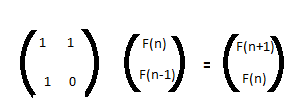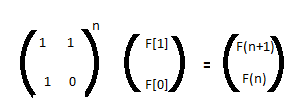Saturday, February 23, 2013

Fibonacci numbers [Matrix exponentiation]

Fibonacci numbers has been a very famous sequence represented as:

F(n) = F(n-1) + F(n-2)
with F(0) = 0, F(1) =1
So what if you need to find the nth term of the series. Its easy to view the recursive nature of the problem.

The code snippet would look something like

```int fib(int n) {
if(n<1) return 0;
if(n == 1) return 1;
return fib(n-1) + fib(n-2);
}
```

Lets try to analyse the sequence of calls for calculating the F(4)Clearly we are computing the same value over and over, and the size of computations will grow exponentially with the increasing value of n. Can we reuse the same value computed before ?

Yes, infact we term this as a technique called memorization or Dynamic programming.
The trick is to store the values as you compute and look up the value before computing it.

The iterative solution to compute the nth Fibonacci is as follows
```int fib(int n) {
if(n < 1)
return 0;
int *a = malloc(sizeof(int) * (n+1));
a = 0; a = 1;
for(int i = 2; i <= n; i++)
a[i] = a[i-1] + a[i-2];

return a[n];
}
```

The above implementation reduces the time complexity to O(n) with a overhead of the O(n) space.

Can we still do better ?
Is it necessary to generate all the previous Fibonacci numbers ?
Let's try to rearrange the formulas to generate the series
F(2) = F(1) + F(0)
F(3) = F(2) + F(1) = 2*F(1) + F(0)
F(4) = F(3) + F(2) = 3*F(1) + 2*F(0)

It appears that every term in the series can be broken down to a form of
F(n) = p*F(1) + q*F(0)

So the task boils down to find the p & q for a particular given 'n'.

Its easy to realize that the subsequent terms can be generated using the matrix multiplication.So we can calculate the nth Fibonacci number asCan we evaluate the power(a,b) in sub-linear time ?
The answer is yes, we will be using the same technique again of reusing the old values computed before.
May be the old computed values concept may not be straightforward.
Lets take a closer look on how to calculate power(a,.b)
power(a, b) = a*a*a*a*.........*a (b times)
can you figure out the overlapping solutions.

We can rewrite the above equation as
power(a,b) = power(a,b/2) * power(a, b/2)           If b is even
power(a,b) = power(a,b/2) * power(a,b/2)  * a     If b is odd

By now you would have realized that the number of multiplications required will be ceil(log(b))
(where log is evaluated in base 2)

Using the same approach we can evaluate the power(matrix M, n)  and number of operations needed will be
(sizeof(M) ^3) * log(n)

In our case it will be 8* log(n)

Hence the nth Fibonacci number can be calculated in O(log(n)). Writing the code snippet for the same is left as an exercise to the reader.
1.